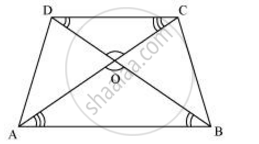# Diagonals of a trapezium ABCD with AB || DC intersect each other at the point O. If AB = 2 CD, find the ratio of the areas of triangles AOB and COD. - Mathematics

Sum

Diagonals of a trapezium ABCD with AB || DC intersect each other at the point O. If AB = 2 CD, find the ratio of the areas of triangles AOB and COD.

#### SolutionSince AB || CD,

∴ ∠OAB = ∠OCD and ∠OBA = ∠ODC (Alternate interior angles)

In ΔAOB and ΔCOD,

∠AOB = ∠COD (Vertically opposite angles)

∠OAB = ∠OCD (Alternate interior angles)

∠OBA = ∠ODC (Alternate interior angles)

∴ ΔAOB ∼ ΔCOD (By AAA similarity criterion)

:.(ar(triangleAOB))/(ar(triangleCOD)) = ((AB)/(CD))^2

Since AB = 2 CD

:. (ar(triangleAOB))/(ar(triangleCOD)) = ((2CD)/(CD))^2 = 4/1 = 4:1

Concept: Areas of Similar Triangles
Is there an error in this question or solution?